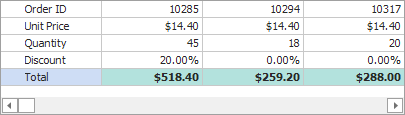20.2 (EAP/Beta)
20.1
19.2
19.1
18.2
18.1
17.2

# RowProperties.UnboundType Property

Gets or sets the data type and binding mode of the row.

Namespace: DevExpress.XtraVerticalGrid.Rows

Assembly: DevExpress.XtraVerticalGrid.v20.1.dll

## Declaration

``````[DefaultValue(UnboundColumnType.Bound)]
[XtraSerializableProperty]
public virtual UnboundColumnType UnboundType { get; set; }``````
``````<DefaultValue(UnboundColumnType.Bound)>
<XtraSerializableProperty>
Public Overridable Property UnboundType As UnboundColumnType``````

## Property Value

Type Default Description
UnboundColumnType

Bound

A UnboundColumnType enumeration value representing the data type and binding mode of the row.

## Remarks

The Vertical Grid Control supports unbound rows. These are rows which aren't bound to any field in the grid's data source, which is specified by the VGridControl.DataSource and VGridControl.DataMember properties. Data for unbound rows should be supplied using one of two methods:

• setting a formula (string expression) to the RowProperties.UnboundExpression property. In expressions, you can refer to other fields, and use various functions and operators. See Expressions, to learn about the syntax.
• handling the VGridControl.CustomUnboundData event. This event can also be used to save any changes made by an end-user in unbound rows via the grid.

To make a row unbound, set its UnboundType property to a UnboundColumnType value that corresponds to the type of data the row will display. For example, if the row is supposed to display integer values, set the UnboundType property to UnboundColumnType.Integer. The data type specified by the UnboundType property determines which editor should be used by default, to edit the row's values. In addition, it also determines how values are aligned within the row, and which validity rules should be applied to this row. See the UnboundColumnType topic, for information on the available data types for unbound rows.

If the row's UnboundType property is set to UnboundColumnType.Bound, it's assumed that this row is not unbound, and it is bound to a specific field in the data source, specified by the RowProperties.FieldName property.

## Examples

Assume that a VGridControl control is bound to the [Order Details] table in the NWind database. The grid contains "Quantity", "UnitPrice" and "Discount" rows which are bound to the corresponding fields in the database table. The example below shows how to add an unbound row to the grid, to display the amount of each order according to the expression: QuantityUnitPrice(1-Discount).

The result is displayed below:``````using DevExpress.XtraVerticalGrid;
using DevExpress.XtraVerticalGrid.Rows;
using DevExpress.Data;
using DevExpress.Utils;
using DevExpress.XtraVerticalGrid.Events;

// Unbound row.
EditorRow rowTotal = null;

private void Form1_Load(object sender, EventArgs e) {
// ...
vGridControl1.CustomUnboundData += new CustomDataEventHandler(vGridControl1_CustomUnboundData);
// Create and initialize the unbound Total row.
rowTotal = new EditorRow();
rowTotal.Properties.Caption = "Total";
rowTotal.Properties.FieldName = "Total";
// Specify format settings.
rowTotal.Properties.Format.FormatType = FormatType.Numeric;
rowTotal.Properties.Format.FormatString = "c";
rowTotal.Properties.ImageIndex = 1;
// Disable editing.
rowTotal.Properties.UnboundType = UnboundColumnType.Decimal;
// Customize the appearance settings.
rowTotal.Appearance.BackColor = Color.LightYellow;
rowTotal.Appearance.Font = new System.Drawing.Font(rowTotal.Appearance.Font,
System.Drawing.FontStyle.Bold);
}

// Provide data for the Total row.
private void vGridControl1_CustomUnboundData(object sender, CustomDataEventArgs e) {
VGridControl vGrid = sender as VGridControl;
if (e.Row == rowTotal && e.IsGetData) {
DataRowView row = (DataRowView)vGrid.GetRecordObject(e.ListSourceRowIndex);
decimal unitPrice = Convert.ToDecimal(row["UnitPrice"]);
decimal quantity = Convert.ToDecimal(row["Quantity"]);
decimal discount = Convert.ToDecimal(row["Discount"]); ;
e.Value = unitPrice * quantity * (1 - discount);
}
}
``````